# The equivalence principle and the deflection of light

### The connection between one of the fundamental principles of general relativity and the gravitational deflection of light

#### An article by Markus Pössel

When Einstein developed his theory of general relativity, one starting point was the so-called equivalence principle. Roughly, it states that an observer in an elevator cannot tell whether he and the elevator are floating in space, far from all sources of gravity, or whether the elevator is in free fall in a gravitational field. In particular, the laws of physics in an elevator in free fall are those of gravity-free space: the laws of special relativity.

Even without much knowledge of general relativity, it is possible to derive some of its main predictions directly from the equivalence principle. One such prediction is that light is influenced by gravity!

## Light and the elevator: the inside view

Consider a falling elevator. More precisely, think about an enclosed room which is like an elevator cabin, but (in contrast with ordinary elevators) which is in free fall. Assume that, at the start of our experiment, the elevator is still at rest. At this exact moment in time, we shoot a brief pulse of laser light into the elevator through a little hole in the elevator’s side. Our laser is aimed in such a way that the light travels horizontally as it enters the elevator. What will the subsequent motion of our light pulse look like?

If you are an observer inside the elevator, then it is easy to find an answer to that question. The elevator is in free fall, and so are you. According to the equivalence principle, the laws of physics in your immediate neighbourhood are those of special relativity: Light travels at a constant speed along straight lines. Therefore, light entering the the cabin horizontally will continue to travel in the horizontal direction. If there is a second hole in the elevator wall, straight across from the first, then the light will leave the cabin through that hole: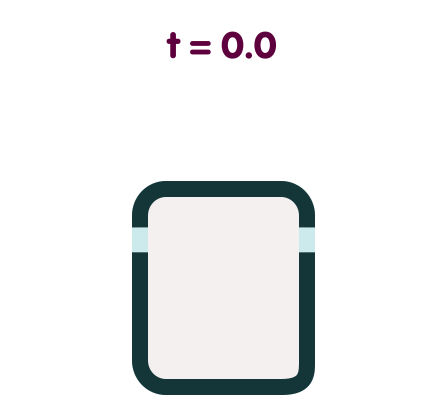In the animation, the laser pulse is the red dot. Light speed has been slowed down artificially so you can follow the dot’s progress; to see that it is travelling along a straight line, the dot has been made to leave a trace.

## Elevator and light: the outside view

Now that we know how light travels as seen by an observer inside the cabin, we can deduce what an outside observer will see.

Initially, the light travels horizontally. On this, both observers agree given that they are at rest relative to each other at the start of the experiment. Also, both observers agree that the light pulse enters through one hole, and leaves through the other. But from the outside observer’s point of view, the elevator starts to fall at the precise moment that the light pulse enters it. Whatever the details of the light pulse’s motion, it will require a certain time to cross the elevator compartment. During that time, the cabin will have moved downward – and so will the second hole, through which the light pulse later makes its exit. Consequently, from the point of view of the outside observer, the light pulse cannot travel along a straight line. After all, a straight line which is initially horizontal remains horizontal and at the same height! Instead, the outside observer will see something like this: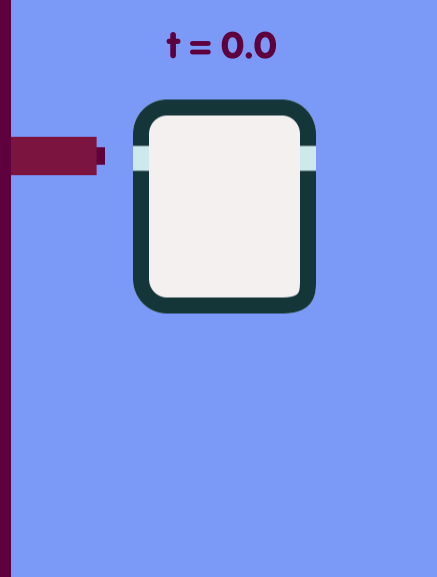The time that it takes for light to cross the cabin is so short that the speed reached by the falling cabin (as judged by the outside observer) is much, much smaller than that of light. Under these circumstances, we need not consult relativity. Without much loss of accuracy, we can describe the cabin’s fall, as well as the parabola which is the orbit of the laser pulse, with the help of classical mechanics.

Since the speed of light in this animation is much lower than in reality, the parabola can readily be seen. For light moving at the proper speed of light, you would not distinguish this parabola from a straight line. Assuming that the two holes in the elevator walls are 1.5 metres (1.64 yards) apart, the light will fall by no more than a tenth of a trillionth of a millimetre as it traverses the cabin – a fraction of the diameter of an atomic nucleus.

## The big picture

Our argument does not require the actual, physical presence of the cabin. Since the cabin and the light never actually interact, our argument must hold even in the absence of a falling cabin. Quite generally, we must conclude thatq light is deflected by gravitation.

Still, there is a fundamental restriction implicit in our use of the equivalence principle: Only in a small region of space, and over a brief time period, can we use the laws of special relativity and end up with a good approximation. Only in a small falling elevator can we assume that light propagates at constant speed, along a straight line.

On the other hand, if we actually want to measure the deflection of light, we will have to look at the big picture. We must consider not just local, but global deflection of light; for instance light from a distant star passing close to the Sun before it reaches an observer here on Earth.

Is it possible to generalize our argument, in order to deduce the global deflection of light? One natural generalization would appear to be the following: Let us divide the region of space traversed by the light into thin strips. In each strip, we imagine a falling elevator cabin to determine how the light is deflected while crossing that particular strip. Patching the strips together, we obtain the total deflection of light which crosses that particular region: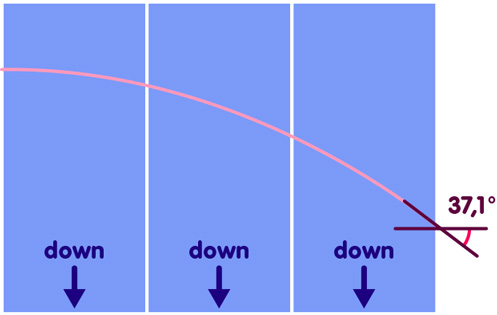There’s a catch, though (or rather: a tacit assumption). With every imaginary falling cabin, we’re tracing the behaviour of light in one single strip. But how do we patch these strips together to reconstruct the whole of space? The answer depends on the geometry of space. In fact, one can define the geometry of space as the answer to the question of how infinitesimally small regions of space join together to form a whole.

The magnitude of the deflection of light depends on the way the strips are joined. In the illustration above, we have tacitly assumed the ordinary, Euclidean geometry taught in high school. There are other possibilities, though. Think of a curved surface – for instance, a gently sloping hill. You cannot cover this hill with square tiles in a regular chessboard pattern. Instead, you will either have to leave gaps between the tiles, or you will have to shape your tiles a bit differently. Similarly, a more complex spatial geometry might force us to shape our strips into little wedges, which are patched together as in the following illustration: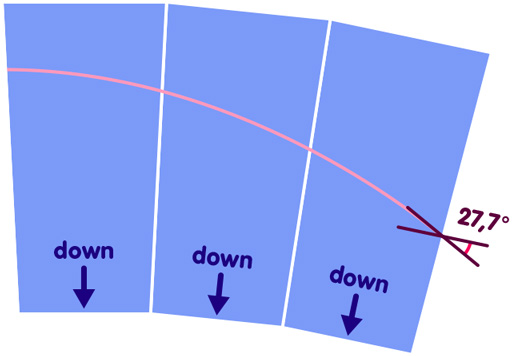For each individual wedge, an arrow shows which way is down – the direction in which the imaginary elevator is falling. Light is bent downward, but in a sense, so is space itself. The result is that, relative to space, the light ray is not bent as much as in the Euclidean case. In other words, the angle between the horizontal direction in the last wedge (which is not the same as the horizontal of the web page, but rather the direction at right angles to “down”) is not as large as in Euclidean geometry.

One could also imagine the opposite case, in which the curvature of space increases the magnitude of light deflection, as sketched here: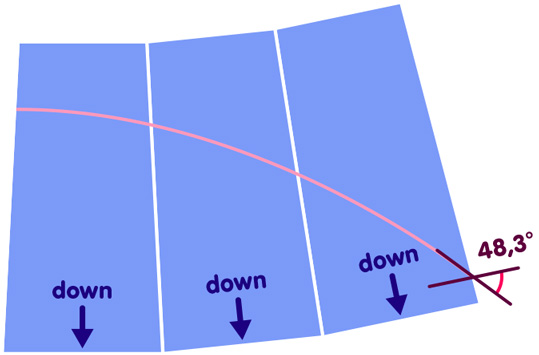In this case, space “bends toward the light”: The horizontal direction and the downward direction change from one strip to the next in such a way that the deflection of the light relative to the local horizontal direction of the last strip is significantly larger than in the Euclidean case.

In consequence, you can only make statements about the large-scale deflection of light if you know the geometry of space.

Before Einstein showed that space geometry and gravity are intimately linked, it was eminently plausible to assume that space geometry is Euclidean. If you make our falling-elevator argument in Euclidean space, you can calculate “Newtonian light deflection” for interesting cases like starlight passing the sun. This deflection is a direct consequence of the equivalence principles.

On the other hand, in Einstein’s theory of general relativity, the presence of a mass curves the surrounding space in a way that is similar to the “bending of space toward the light” in our last example above. The resulting angle of deflection for light passing close to the Sun is exactly twice as large as in the Newtonian case – with the equivalence principle and the curvature of space each responsible for half of that value.

The case in which the deflection of light is diminished by the curvature of space is of historical interest only: At the same time that Einstein wrote his first articles on what is now called general relativity, the Finnish physicist Gunnar Nordström looked for different ways to construct a theory of gravity which was compatible with special relativity. The model known to historians of science as “Nordström’s second theory” incorporates the equivalence principle, but space is curved in exactly the right way to cancel out local light deflection.

All in all, the equivalence principle provides a powerful argument that light is influenced by gravity. In fact, it was through such reasoning that Einstein first thought about the deflection of light as he was working on general relativity. However, if we want to be certain whether or not there is large-scale light deflection, we need to go beyond the equivalence principle, and take into account the geometry of space.

## Further Information

This spotlight text supplements the chapter General relativity of Elementary Einstein.

For basic information about the equivalence principle, we recommend the spotlight text The elevator, the rocket, and gravity. Related spotlight texts can be found in the category General relativity.

More technical information about the crucial difference between local and global light deflection can be found in the article

J. Ehlers and W. Rindler, “Local and Global Light Bending in Einstein’s and other Gravitational Theories” in General Relativity and Gravitation 29 (1997), p. 519-529.

###### Colophon
Markus Pössel

is the managing scientist at Haus der Astronomie, the Center for Astronomy Education and Outreach in Heidelberg, and senior outreach scientist at the Max Planck Institute for Astronomy. He initiated Einstein Online.